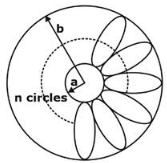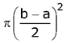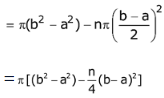# GATE Solved Paper 2020 - GATE 2020

• A

90• B

80• C

40• D

110• Option : A
• Explanation :
P = 31
Q = 32 = 9
R = 33 = 27
S = 34 = 81
T = 35 = 243
So, Q + S = 9 + 81 = 90

• A

1800• B

600• C

1200• D

900• A

good• B

praiseworthy• C

desirable• D

extremely poor• Option : D
• Explanation :
His knowledge of the subject was excellent, but his classroom performance was extremely poor.

• Option : B
• Explanation :
Billions of people are affected by melting glaciers.

• Option : B
• Explanation :Radius of inner circle = a
Radius of outer circle = b
Radius of small circle = (b - a)/2
area of donut shaped figure = π(b2 – a2)
Area of each small circle =total area of all small circle= nArea of remaining portionRelated Quiz.
GATE 2020